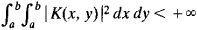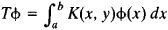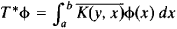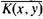[′aj‚ȯint ′äp·ə‚rād·ər]
(mathematics)
An operator B such that the inner products (Ax,y) and (x,By) are equal for a given operator A and for all elements x and y of a Hilbert space. Also known as associate operator; Hermitian conjugate operator.
McGraw-Hill Dictionary of Scientific & Technical Terms, 6E, Copyright © 2003 by The McGraw-Hill Companies, Inc.
The following article is from The Great Soviet Encyclopedia (1979). It might be outdated or ideologically biased.

a concept of operator theory. Two bounded linear operators T and T* on a Hilbert space H are said to be adjoint if, for all vectors x and y in H,

(Tx, y) = (x, T*y)

For example, ifthen the adjoint of the operatoriswhereis the complex conjugate of the function K(x, y).

If T is not bounded and if its domain of definition Dm is everywhere dense (seeDENSE AND NONDENSE SETS), then the adjoint of T is defined on the set of vectors y for which a vector y* can be found such that the equality (Tx, y) = (x, y*) holds for all xDm. It is assumed here that T*y = y*.

The concept of adjoint operator can be extended to operators in other spaces.

References in periodicals archive ?
where [mathematical expression not reproducible] denotes the orthogonal projection in [L.sup.2]([OMEGA]) onto the admissible set of the control and [B.sup.*] is the adjoint operator of B.
The matrix-vector multiplication should be replaced with the specifically designed function to perform the equivalent of the forward operator [g.sub.A] and adjoint operator [g.sup.H.sub.A].
The main properties of the adjoint operator were stated in Ch.
A : [H.sub.1] [right arrow] [H.sub.3] is a bounded linear operator with its adjoint operator [A.sup.*], [sigma] [member of] (0,1) is a parameter controlling step length, 0 < [gamma] < [eta] < 1/(1 + [square root of (1 + [L.sup.2])]), and {[[alpha].sub.n]} [subset] (0,1).
Throughout the paper, we will assume that A(t) is invertible, A(t) = [A.sup.*](t) for all [mathematical expression not reproducible] for all t [member of] [q.sup.N], where * denotes the adjoint operator. Note that we can also define the operator L using the infinite matrix
We use the same notation H to denote this self adjoint operator. The operator admits the partial wave expansion.
PA = A[P.sup.*] where [P.sup.*] is the adjoint operator of P with respect to [<x, x>.sub.H], defined by [P.sup.*]x = x - U[<U, AU>.sup.-1.sub.H][<AU,x>.sub.H].
(i) [??] (ii) Let X and Y be left Banach G-modules and suppose that T : X [right arrow] Y is a bounded linear G-module map and [T.sup.*] : [Y.sup.*] [right arrow] [X.sup.*] is its adjoint operator. For [mu] [member of] M(G), x [member of] X and [phi] [member of] [Y.sup.*],
It is claimed that the mapping f is contraction with constant [beta] = 1/2, A is a bounded linear operator on R with adjoint operator [A.sup.*] and [parallel]A[parallel] = [parallel][A.sup.*] [parallel] = 1/2, and B is a strongly positive bounded linear self-adjoint operator with constant [bar.[gamma]] = 1 on R.
In the theory of inverse problems, we are asked to find the solution of the operator equation Bf = g where B: H [right arrow] H is a self adjoint operator on a Hilbert space H, and g e H is the exact datum.
Recall that the adjoint operator [T.sup.*] is then defined by [T.sup.*][phi] = [[phi].sup.*] for all [phi] [member of] D([T.sup.*]).

Site: Follow: Share:
Open / Close